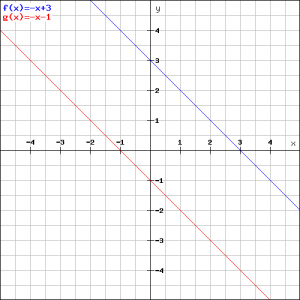# Solution assignment 05 Linear functions and graphs

### Assignment 5

Draw the graphs of the following linear functions: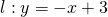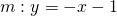and determine the intersection point of the lines.

### Solution

A quick look at both equations shows that they have equal slopes: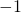and thus the lines are either parallel or coincide. Because the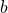-values are different (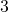and, respectively), the lines are parallel and thus do not intersect. See the figure, and identify which line corresponds with which equation.